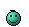# MATH TIME!

Discussion in 'User Topics' started by Mr.Lame, Jan 9, 2018.

1. Okay, suppose we are given a parabolic function in the form:

y = a(x - h)^2 + k, where a ≠ 0

The vertex (for a parabola, this is the turning point) will be at (h,k). If a < 0 then the parabola will open downwards and the vertex will be at the maximum, but if 0 < a then the parabola opens upwards and the vertex will be at the minimum.

The parabola we've been given may be written as:

y = 1(x - 0)^2 + (-7)

This means the vertex is at (0,-7) and the parabola opens upwards, so the minimum value is -7. Let's look at a graph of the function:The blue curve is the parabola, and the dots on the curve represent the 4 smallest values of the parabola when x is an integer. The horizontal lines represent the value of the parabola for those integral values of x, namely:

y = -7
y = -6
y = -3
y = 2

Does that make sense?

•Love x 1
2. ### Mr.LameVeteran

Blogs:
28
Joined:
Dec 7, 2009
Posts:
1,540
628
Yes I remember this!!!!! so in other words I should go to my calculator for this type of question as well correct?

•Like x 1
3. You shouldn't need a calculator for this, except perhaps if you want to graph the function to get an idea what the curve looks like and how it behaves.

•Like x 1
4. ### Mr.LameVeteran

Blogs:
28
Joined:
Dec 7, 2009
Posts:
1,540
628
Hey @MarkFL, I'm having some issues again with ABS

I'm trying to understand why there is a minus instead of a plus |x-4|

The distance between x and 4 is greater than 0 but less than 4.

Is it because it's less than four?

0<|x-4|<4

•Like x 1
5. We've got two cases to consider here...the first is:

x - 4 ≥ 0

Recall that when |x| = x when x ≥ 0, and |x| = -x when x < 0.

In this case, we can write:

0 < x - 4 < 4

4 < x < 8

The second case is:

x - 4 < 0

In this case we may write:

0 < 4 - x < 4

Multiply through by -1:

0 > x - 4 > -4

4 > x > 0

And so our solution, in interval notation, is:

(0, 4) U (4, 8)

On the number line, this is:•Winner x 1
6. ### Mr.LameVeteran

Blogs:
28
Joined:
Dec 7, 2009
Posts:
1,540
628
That part makes sense I just don't understand why it's a x-4 :/ instead of x+4

7. ### Mr.LameVeteran

Blogs:
28
Joined:
Dec 7, 2009
Posts:
1,540
628
Thought abs was suppose to be positive inside!

8. The distance between x and 4 is the magnitude of the difference between x and 4. For a difference we use subtraction, and for a magnitude we use absolute value, thus the distance d between x and 4 on the number line is:

d = |x - 4|

•Love x 1
9. ### Mr.LameVeteran

Blogs:
28
Joined:
Dec 7, 2009
Posts:
1,540
628
Interesting was trying to solve got everything except mixed up the sign and didn't know why T.T

10. No, the actual absolute value is never negative, we always have:

0 ≤ |x|

But the value of x itself can be any real number. If x is negative then:

|x| = -x

If x is non-negative, then:

|x| = x

For example, if we have:

|-2|

Then we see -2 < 0 and so:

|-2| = -(-2) = 2

•Love x 1
11. ### Mr.LameVeteran

Blogs:
28
Joined:
Dec 7, 2009
Posts:
1,540
628
Sweet. I'll have more for you soon! Hahaha

•Love x 1
12. ### Mr.LameVeteran

Blogs:
28
Joined:
Dec 7, 2009
Posts:
1,540
628
With your help @MarkFL I feel like I can obtain my 4.0 GPA•Winner x 1
13. ### Mr.LameVeteran

Blogs:
28
Joined:
Dec 7, 2009
Posts:
1,540
628
@MarkFL Okay my brain hurts I feel like I'm making a small error.

Simplify the variable equation:
3/4(5a+2)-1/2(3a-5)

Now I found the LCD to get rid of the fractions but I feel that's not what I was suppose to do... (I have the answer). helppp meeee pleassseee <3

14. ### Mr.LameVeteran

Blogs:
28
Joined:
Dec 7, 2009
Posts:
1,540
628
Nope, it's fractions on the outside of the brackets! Where do you get this images? I'll capture the questions lol

•Winner x 1
15. ### Mr.LameVeteran

Blogs:
28
Joined:
Dec 7, 2009
Posts:
1,540
628
Snap that was awesome, yeah it's 9/4a+4

•Love x 1
16. ### Mr.LameVeteran

Blogs:
28
Joined:
Dec 7, 2009
Posts:
1,540
628
So I almost had it right I should have just timesed the fractions instead of everything.

17. Yeah, if you distribute the 1/4 then you do get (9/4)a + 4.I actually prefer the first form I gave.•Winner x 1

Blogs:
28
Joined:
Dec 7, 2009
Posts:
1,540